Click to Chat

1800-1023-196

+91-120-4616500

CART 0

• 0

MY CART (5)

Use Coupon: CART20 and get 20% off on all online Study Material

ITEM
DETAILS
MRP
DISCOUNT
FINAL PRICE
Total Price: Rs.

There are no items in this cart.
Continue ShoppingElectrode and Cell Potentials

Table of Content

EMF of A Galvanic Cell

Difference between EMF and Potential Difference

Nernst Equation

Potential of Single Electrode (Anode)

Potential at Copper Electrode (Cathode)

Concept of Equilibrium in Electrochemical Cell

Related Resources

EMF of A Galvanic CellEvery galvanic or voltaic cell is made up of two half-cells, the oxidation half-cell (anode) and the reduction half-cell (cathode). The potentials of these half-cells are always dif­ferent. On account of this difference in electrode potentials, the electric current moves from the electrode at higher potential to the electrode at lower potential, i.e., from cathode to anode. The direction of the flow of electrons is from anode to cathode.The difference in potentials of the two half-cells is known as the electromotive force (EMF) of the cell or cell potential.

The emf of the cell or cell potential can be calculated from the values of electrode potentials of the two half-cells constitut­ing the cell. The following three methods are in use:

When oxidation potential of anode and reduction poten­tial of cathode are taken into account:

ECello = Oxidation potential of anode + Reduction potential of cathode

ECello  = Eoxo (anode) + Eredo (cathode)

When reduction potentials of both electrodes are taken into account:

ECello = Reduction potential of cathode - Reduction potential of anode

ECello = ECathodeo - EAnodeo  = Erighto - Elefto

When oxidation potentials of both electrodes are taken into account:

ECello  = Oxidation potential of anode - Oxidation potential of cathode

ECello  =  Eoxo (anode) - Eredo (cathode)Difference between EMF and Potential Difference

The potential difference is the difference between the electrode potentials of the two electrodes of the cell under any condition while emf is the potential generated by a cell when there is zero electron flow, i.e., it draws no current. The points of difference are given below:

S.No.

EMF

Potential Difference

1

t is the potential difference between two electrodes when no current is flowing in the circuit.

It is the difference of the electrode potentials of the two electrons two electrodes when the cell is under operation.

2

It is the maximum voltage that the cell can deliver.

It is always less than the maximum the cell can deliver.

3

It is responsible for the steady flow of current in the cell.

It is not responsible for the steady flow of current in the cell.

Nernst Equation

The electrode potential and the emf of the cell depend upon the nature of the electrode, temperature and the activities (concentrations) of the ions in solution. The variation of electrode and cell potentials with concentration of ions in solution can be obtained from thermodynamic considerations. For a general reaction such as

M1A + m2B .....  n1X + n2Y + ....   .......(i)

Occurring in the cell, the Gibbs free energy change is given by the equation

where

'a' represents the activities of reactants and products under a given set of conditions and

?Go refers to free energy change for the reaction when the various reactants and products are present at standard conditions.

The free energy change of a cell reaction is related to the electrical work that can be obtained from the cell, i.e.,

?Go = -nFEcell and ?Go = -nFEo.

On substituting these values in Eq. (ii) we get

This equation is known as Nernst equation.

Putting the values of

R=8.314 JK-1 mol-1,

T = 298 K and

F=96500 C,

Eq. (iv) reduces to

Potential of Single Electrode (Anode)

Consider the general oxidation reaction,

M →  Mn+ + ne-

Applying Nernst equation,

where Eox is the oxidation potential of the electrode (anode),  is the standard oxidation potential of the electrode.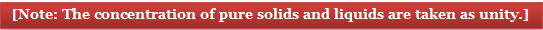Let us consider a Daniell cell to explain the above equations. The concentrations of the electrolytes are not 1 M.

Zn(s)+Cu2+(aq)  Zn2+(aq) + Cu(s)

Cell Representation: Zn(s)|Zn2+(aq)||Cu2+(aq)|Cu

Potential at zinc electrode (Anode)

Potential at Copper Electrode (Cathode)

Emf of the cell

Ecell = Eox + Ered

The value of n = 2 for both zinc and copper.

Let us consider an example, in which the values of n for the two ions in the two half-cells are not same. For example, in the cell

Cu|Cu2+||Ag+|Ag

The cell reaction is

Cu(s) + 2Ag+ →  Cu2+ + 2Ag

The two half-cell reaction are:

Cu → Cu2+ + 2e-

Ag+ + e- →  Ag

The second equation is multiplied by 2 to balance the number of electrons.

2Ag+ + 2e- →  2 Ag

Ecell = Eox + Ered

All the EMFs that have been used are reduction potentials in volts. Whatever be the value of reduction potentials, substitute them as such, taking into account the signs.

Concept of Equilibrium in Electrochemical Cell

In an electrochemical cell a reversible redox process takes place, e.g., in Daniell cell:

Zn(s)+Cu2+(aq)  Zn2+(aq)+Cu(s)

At equilibrium mass action ratio becomes equal to equilibrium constant,i.e., Q = Ke

Oxidation potential of anode = -Reaction potential of cathode

i.e., EMF = oxidation potential of anode + Reduction potential of cathode = 0

Cell is fully discharged

According to Nernst equation:

E = Eo - 0.0591/n log10 Q at 25o C

At equilibrium, E = 0, Q = K

0 = Eo 0.0591/n log10 K

K = Antilog [(nEo)/0.0591]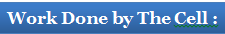Let n faraday charge be taken out of a cell of emf E; then work done by the cell will be calculated as:

Work = Charge x Potential = nFE

Work done by the cell is equal to decrease in free energy.

-?G = nFE

Similarly, maximum obtainable work from the cell will be

Wmax = nFE°

where, Eo = standard emf or standard cell potential.

-?G = nFE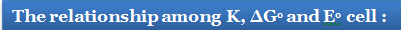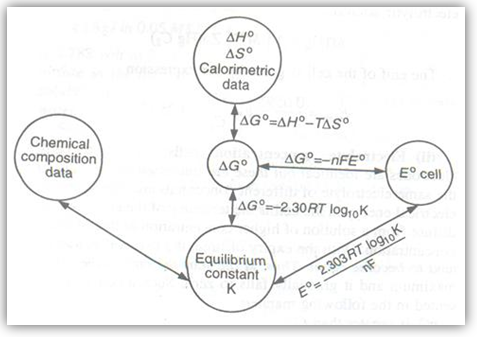Let n Faraday charge flows out of a cell of emf E,

Then -?G = nFE                      ...... (i)

Gibbs-Helmholtz equation from thermodynamics may be given as

?G = ?H + T (∂?G/∂T)P         ...... (ii)

From equation (i) and (ii) we get

-nFE = ?H + T (∂(-nFE)/∂T)P = ?H-nFT(∂E/∂T)P

?H = -nFE + nFT(∂E/∂T)P

Here (∂E/∂T)P = Temperature coefficient of cell

Case I:   When (∂E/∂T)P = 0, then ?H= -nFE

Case II: When (∂E/∂T)>0, then nFE>?H, i.e., process inside the cell is endothermic.

Case III:  When (∂E/∂T)<0 , then nFE<?H, i.e., process inside the cell is exothermic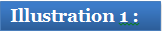The emf of the following cell was 0.2699 V at 293 K and 0.2669V at 303 K

Pt | H2 | HCl (aq) || Hg2Cl2 | Hg

Calculate (i) temperature coefficient

(ii) dG, dH and dS at 293 K

Solution:

(i) Temperature coefficient = dEcell/dT  =(0.2669-0.2669)/(303-293) VK-1 = -3  10–4 VK–1  = -3  10–4 VK–1

(ii) at 293 K

dG = –nFEcell [Q Ecell at 293 K = 0.2699 volt]

= – 2  96,500  0.2699 J  = – 52.09 kJ

dH293K = nF[T(dE/dT)-Ecell]

= 2  96,500 [293  -3  10–4 – 0.2699]   = – 69.055 KJ

(iii) dSat 293 K = nF(dS/dT)p = 2 96,500  – 3  10–4 = – 57.9 JK–1Question 1:

Which of the following expressions for cell potential is incorrect?

a. ECello = Oxidation potential of anode + Reduction potential of cathode

b. ECello  = Eo (anode) + Eo (cathode)

c. ECello =  Erighto- Elefto

d.

Question 2:

Consider the reaction,

2Ag+ + Cd →   2Ag + Cd2+

The standard electrode potentials for Ag+ →  Ag and Cd2+→  Cd couples are 0.80 volt and -0.40 volt, respectively.

What is the standard potential Eo for this reaction?

a. 1.20  V

b. 12.0 V

c. 120 V

d. 0.12 V

Question 3:

What will be the emf of the following cell

Zn|Zn2+(aq)(1.0M)||Cu2+(aq)(1.0M)|Cu

The standard electrode potentials are

Cu2+ + 2e- →  Cu(aq)                 Eo = 0.350 volt

Zn2+ + 2e- →  Zn(aq)                   Eo = -0.763 volt

a. 1.113 V

b. 12.43 V

c. 1.92 V

d. 5.324 V

Question 4:

What will be  the electrode potential at a copper electrode dipped in a 0.1 M solution of copper sulphate at 25 oC?

The standard electrode potential of Cu2+/Cu system is 0.34 volt at 298 K.

a. 10.113 V

b. 0.31045 V

c. 11.92 V

d. 9.324 VQ.1

Q.2

Q.3

Q.4

c

a

a

b

Related Resources

Look into the Sample Papers for an idea about type of questions asked in IIT JEE.

You can also refer to Electrode Potential

To read more, Buy study materials of Electrochemistry comprising study notes, revision notes, video lectures, previous year solved questions etc. Also browse for more study materials on Chemistry here.### Course Features

• 731 Video Lectures
• Revision Notes
• Previous Year Papers
• Mind Map
• Study Planner
• NCERT Solutions
• Discussion Forum
• Test paper with Video Solution